###### eISSN: 2641-936X# Electrical & Electronic Technology Open Access JournalCorrespondence:

Received: January 01, 1970 | Published: ,

Citation: DOI:

# Abstract

Complementary Metal Oxide Semiconductor (CMOS) is normally used to describe small measure of memory on a Computer motherboard that stores the Basic Input and Output Settings (BIOS) settings. Some of these BIOS settings include the system time and date and in hardware settings. CMOS is also used for constructing integrated circuits. It is normally used for microprocessors, micro controller and other digital circuits. In this review paper of Nano CMOS, its VI characteristics, data analysis and performances are discussed and graphs are depicted.

Keywords: nano, nanotechnology, nano CMOS

# Introduction

Complementary Metal Oxide Semiconductor (or simply called as CMOS) is used to design various logic circuits such as switches, inverter, transmission, NAND, NOR, XOR gates including compound logic, Static Random Access Memory (SRAM) Cells, registers etc. There are N-type and P-type transistors. P-type uses electrons and N-type uses holes. The gate of CMOS is made of aluminum alternatively, polysilicon can be used. Silicon dioxide is the substantial between gate and channel. Semiconductor material is mostly silicon alternatively, GaAs can also be used based on the applications and design.1

Unlike any other BIPOLAR circuits, a Complementary MOS circuit has almost no static power scattering. Power is just dissipated if circuit switches "ON" or "OFF". This allows integrating more CMOS logic gates on an IC than bipolar technologies, resulting better performance. Complementary Metal Oxide Semiconductor transistor comprises of P-channel MOS (PMOS) and N-channel MOS (NMOS) (Figure 1).2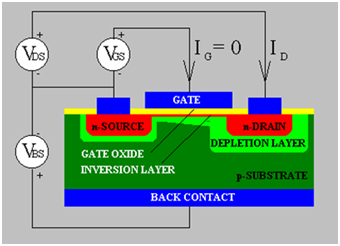Figure 1 Depicts traditional CMOS transistor.

Nano complementary metal oxide semiconductor

Carbon nanotubes can be metallic or semiconductor based on their chirality (Figure 2–4).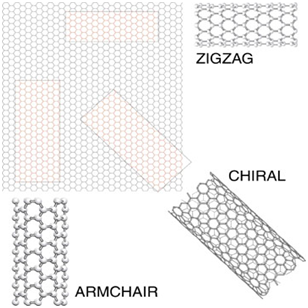Figure 3 Depicts Chirality of Carbon nanotube.Figure 4 Depicts construction of complementary metal oxide semiconductor using carbon nanotubes.

Characteristics of nano complementary metal oxide semiconductor3,4 (Figure 5–12).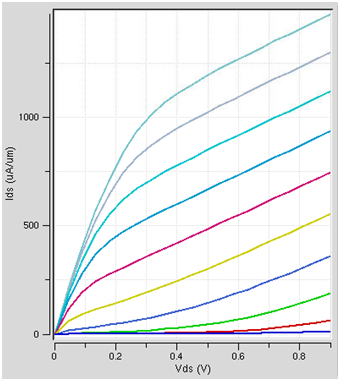Figure 5 Depicts the graph of N type for 32nm size (Ids vs Vds).Figure 6 Depicts the graph of N type for 32nm (Ids vs Vgs).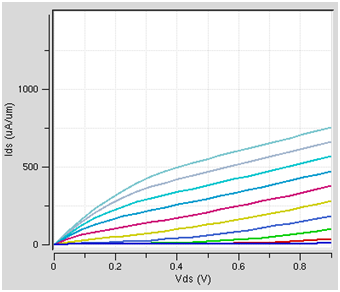Figure 7 Depicts the graph of P type for 32nm (Ids vs Vds).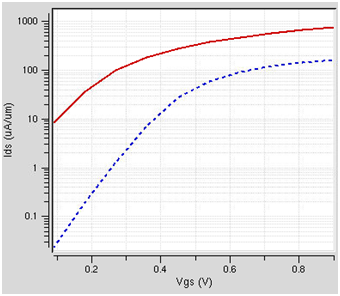Figure 8 Depicts the graph of P type for 32nm (Ids vs Vgs).Figure 9 Depicts the graph of N type for 45nm (Ids vs Vds).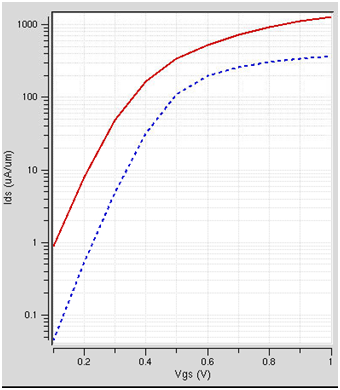Figure 10 Depicts the graph of N type for 45nm (Ids vs Vgs).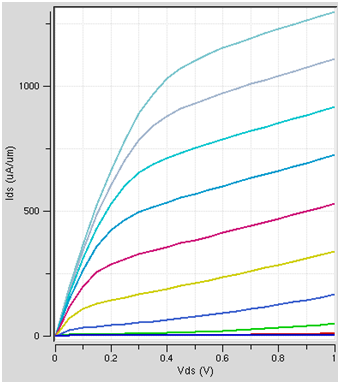Figure 11 Depicts the graph of P type for 45nm (Ids vs Vds).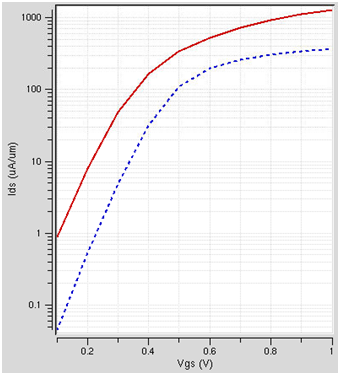Figure 12 Depicts the graph of P type for 45nm(Ids vs Vgs).

Data analysis

Data Analysis was performed for N-Type and P-Type for various voltages (0.01v, 25v, 50v) and performances for (ID vs VDS) and (ID vs VGS) are plotted (Figure 13–24).Figure 13 Depicts the graph of N type (ID vs VDS) at 0.01 V.Figure 14 Depicts the graph of N type (ID vs VGS) at 0.01 V.Figure 15 Depicts the graph of P type (ID vs VDS) at 0.01 V.Figure 16 Depicts the graph of P type (ID vs VGS) at 0.01 V.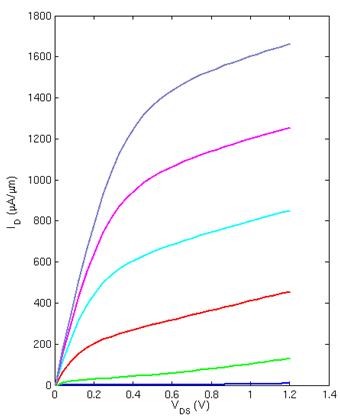Figure 17 Depicts the graph of N type (ID vs VDS) at 25 V.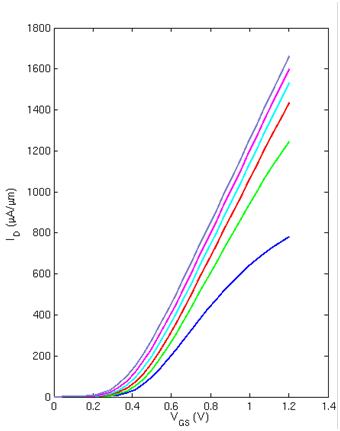Figure 18 Depicts the graph of N type (ID vs VGS) at 25 V.Figure 19 Depicts the graph of P type (ID vs VDS) at 25 V.Figure 20 Depicts the graph of P type (ID vs VGS) at 25 V.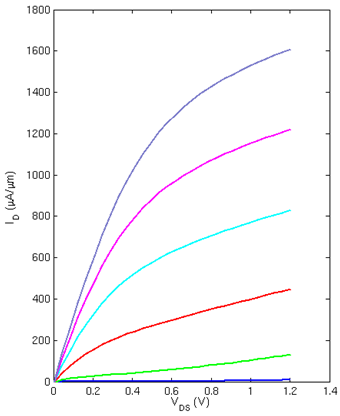Figure 21 Depicts the graph of N type (ID vs VDS) at 50 V.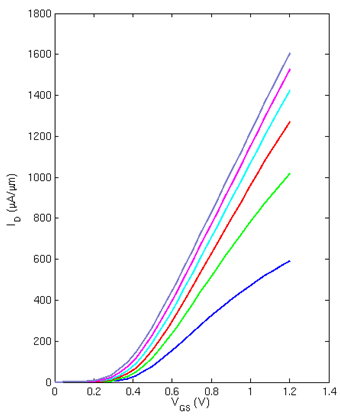Figure 22 Depicts the graph of N type (ID vs VGS) at 50 V.Figure 23 Depicts the graph of P type (ID vs VDS) at 50 V.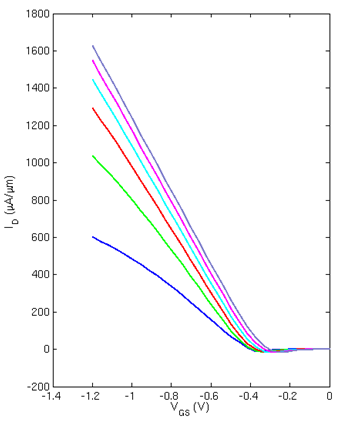Figure 24 Depicts the graph of P type (ID vs VGS) at 50 V.

# Results

A.     Interpretation of Graphs

In Figure 14 (ID vs VGS) the purple color graph, current (ID) has a sudden increase in spike and has steep fall and maintains constant at 1400 (approx.) because of very low voltage (0.01V).

In Figure 15 (ID vs VGS) the blue color graph, current (ID) has a sudden slope and raises it and maintains constant at 1500 (approx.) because of very low voltage (0.01V).

# Conclusion

In this review paper what is claimed are:

VI Characteristics of nano complementary metal oxide semi-conductor for an N Type and P Type (32nm) (Ids vs Vds) are plotted.

VI Characteristics of nano complementary metal oxide semi-conductor for an N Type and P Type (32nm) (Ids vs Vgs) are plotted.

VI Characteristics of nano complementary metal oxide semi-conductor for an N Type and P Type (45nm) (Ids vs Vds) are plotted.

VI Characteristics of nano complementary metal oxide semi-conductor for an N Type and P Type (45nm) (Ids vs Vgs) are plotted.

Data Analysis of nano complementary metal oxide semi-conductor for an N Type and P Type (ID vs VDS) at 0.01V.

Data Analysis of nano complementary metal oxide semi-conductor for an N Type and P Type (ID vs VGS) at 0.01V.

Data Analysis of nano complementary metal oxide semi-conductor for an N Type and P Type (ID vs VDS) at 25V.

Data Analysis of nano complementary metal oxide semi-conductor for an N Type and P Type (ID vs VGS) at 25V.

Data Analysis of nano complementary metal oxide semi-conductor for an N Type and P Type (ID vs VDS) at 50V.

Data Analysis of nano complementary metal oxide semi-conductor for an N Type and P Type (ID vs VGS) at 50V.

# Acknowledgements

Author would like to thank Prof. Navarun Gupta, Prof. Hassan Bajwa, Prof. Linfeng Zhang and Prof. Hmurcik for their academic support. Author also thanks anonymous reviewers for their comments.

# Conflict of interest

The author declares there is no conflicts of interest.